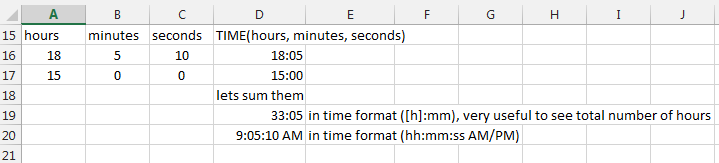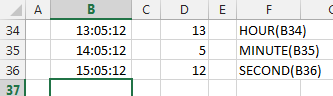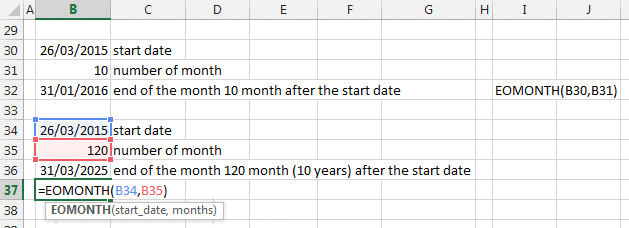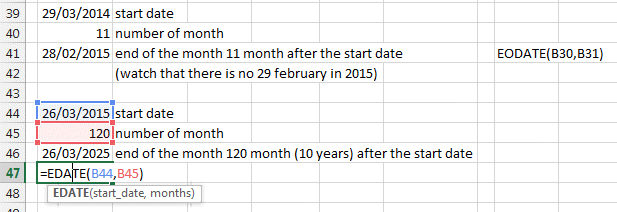## Time Functions in Excel (part2)

Here some more of the very important date and time function of Excel that allow you to make useful formulas that give you the result you want.

Look at part 1 for Date functions here.

Part 3 deals with weeks, weekdays and years.

Two very useful functions are the NETWORKDAYS and WORKDAY to calculate difference between date without the weekends and holidays as well as find the next working day after a defined date. These are part of the top 10 functions in Excel. Find an explanation in our top 10 page.

### TIME, HOUR, MINUTE, SECOND functions

Same as for the Day, Month and Year function, HOUR, MINUTE and SECOND allow you to play with time. Who does not want to play with time (;-).

Controlling time, everyones dream! With Excel you CAN.

First TIME(hours, minutes, seconds) will create a time value from 3 numbers. Like the DATE function.The Formating of time is very important if you want for example to show it in AM/PM format or 24Hours format.

HOURS, MINUTE, SECOND

Here how to get the hour, minute and seconds out of a time value.Hour(Cell value)

Minute(cell value)

Second(cell value)

### EDATE, EOMONTH

These two functions are used to calculate the date after a certain number of month or the end of the month after a certain number of months.

EOMONTH(Start date, number of month after)EDATE(Start date, number of month after)

EDATE is very useful to calculateHere you will find listed all the Date and Time functions so that you can have a good overview. In Bold are the ones most used and useful.

### All the Date and Time functions

DATE: The serial number of a particular date/p>

DATEVALUE: Converts a date in the form of text to a serial number

DAY: Converts a serial number to a day of the month

DAYS360: Calculates the number of days between two dates based on a 360-day year/p>

EDATE: The serial number of the date that is the indicated number of months before or after the start date

EOMONTH: The serial number of the last day of the month before or after a specified number of months

HOUR: Converts a serial number to an hour

MINUTE: Converts a serial number to a minute

MONTH: Converts a serial number to a month

NETWORKDAYS: The number of whole workdays between two dates

NOW: The serial number of the current date and time

SECOND: Converts a serial number to a second

TIME: The serial number of a particular time

TIMEVALUE: Converts a time in the form of text to a serial number

TODAY: The serial number of today's date

WEEKDAY: Converts a serial number to a day of the week

WEEKNUM: Converts a serial number to a number representing where the week falls numerically with a year

WORKDAY: The serial number of the date before or after a specified number of workdays

YEAR: Converts a serial number to a year

YEARFRAC: The year fraction representing the number of whole days between start_date and end_date

So that's it for the Date and Time Formulas and Functions in Excel.

Now, let's use them....